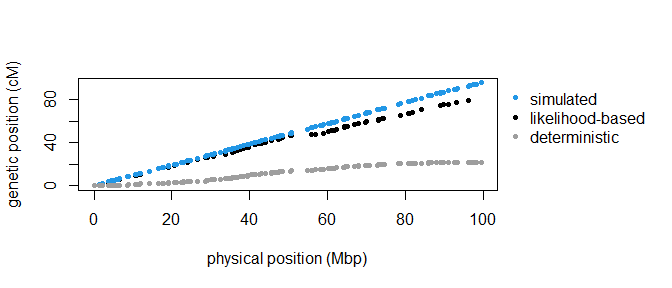# Description of the workflow for constructing a genetic map using hsrecombi

## Introduction

hsrecombi can approximate the genetic-map positions of genetic markers from SNP genotypes in half-sib families. It is referred to Hampel et al. (2018, Fron Gen) and Qanbari & Wittenburg (2020, Genet Sel Evol) for more information on the methodology. The workflow relies on paternal half-sib families but it can be adapted to maternal half-sib families with minor modifications.

In Part I, genotypic data from progeny and their common parents are simulated with the R package AlphaSimR and reshaped into the PLINK format. If genotypic data are already available in the required PLINK format, the workflow starts directly at Part II.

Required input files:

• map files for each chromosome map_chr<chr>.map
• data files for each chromosome hsphase_input_chr<chr>.raw

Note: A pipeline is available at github.

library(hsrecombi)
library(AlphaSimR)
#> Loading required package: R6
library(doParallel)
#> Loading required package: foreach
#> Loading required package: iterators
#> Loading required package: parallel
library(ggplot2)
library(rlist)
Sys.time()
#>  "2021-03-23 08:57:30 CET"

### Global variables

# number of chromosomes
nchr <- 2
# Number of simulated SNPs (e.g., p = 1500 resembles an average bovine chromosome)
p <- 150
# Number of simulated progeny in each half-sib family
n <- 1000
# Directory for (simulated) data
path.dat <- "data"
dir.create(path.dat, showWarnings = FALSE)
# Directory for output
path.res <- "results"
dir.create(path.res, showWarnings = FALSE)
# Number of computing clusters allocated
nclust <- 2

For instance, executing the following workflow with $$p=1500$$ and $$n\geq 1000$$ requires about 120 Mb storage space and 10 min computing time (2.6 GHz processor). With $$p=150$$, it takes about 7 Mb and 20 sec.

## Part I: Data simulation and preparation

### 1: Simulation of genetic data with R package AlphaSimR

founderPop <- runMacs2(nInd = 1000, nChr = nchr, segSites = p)
SP <- SimParam$new(founderPop) SP$setSexes("yes_sys")
# Enable tracing location of recombination events
SP$setTrackRec(TRUE) pop <- newPop(founderPop) N <- 10 ntotal <- N * n my_pop <- selectCross(pop = pop, nFemale = 500, nMale = N, use = "rand", nCrosses = ntotal) probRec <- list() for (chr in 1:nchr) { co.pat <- matrix(0, ncol = p, nrow = ntotal) for (i in 1:ntotal) { if (nrow(SP$recHist[[1000 + i]][[chr]][]) > 1) {
# 1. line contains 1 1 by default
loci <- SP$recHist[[1000 + i]][[chr]][][-1, 2] co.pat[i, loci] <- 1 } } probRec[[chr]] <- colMeans(co.pat) } save(list = c("SP", "founderPop", "pop", "my_pop", "ntotal", "probRec"), file = "data/pop.RData") Two chromosomes, each with $$p$$ SNPs and 1 Morgan length, are simulated. The founder population consists of 1,000 animals with equal gender distribution. The follow-up generation consists of $$N$$ paternal-half sib families with equal number of progeny $$n$$. The study design with the equal number of progeny per family was preferred here for simplicity but this is not required in the subsequent steps. ### 2: Selection of sire haplotypes and progeny genotypes PAT <- my_pop@father rown <- paste(rep(unique(PAT), each = 2), c(1, 2), sep = "_") H.pat <- pullSegSiteHaplo(pop)[rown, ] X <- pullSegSiteGeno(my_pop) # Physical position of markers in Mbp map.snp <- lapply(founderPop@genMap, function(z) z * 100) Note that founderPop@genMap includes genetic positions in Morgan units but physical positions are required in the next steps. ## Part II: Main analysis cl <- makeCluster(nclust) registerDoParallel(cl) ### Steps 1-5: Calculation of pairwise recombination rates out <- foreach(chr = 1:nchr, .packages = "hsrecombi") %dopar% { # 1: Physical map map <- read.table(file.path(path.dat, paste0("map_chr", chr, ".map")), col.names = c("Chr", "Name", "locus_Mb", "locus_bp")) map$SNP <- 1:nrow(map)
locus_Mb <- map$locus_Mb # 2: Genotype matrix genomatrix <- data.table::fread(file.path(path.dat, paste0("hsphase_input_chr", chr, ".raw"))) X <- as.matrix(genomatrix[, -c(1:6)]) # 3: Assign daughters to sire IDs daughterSire <- genomatrix$PAT

# 4: Estimate sire haplotypes and format data
hap <- makehappm(unique(daughterSire), daughterSire, X)
save("hap", file = file.path(path.res, paste0("hsphase_output_chr", chr, ".Rdata")))

# Check order and dimension
io <- sapply(1:nrow(map), function(z) {
grepl(x = colnames(X)[z], pattern = map$Name[z]) }) if (sum(io) != nrow(map)) stop("ERROR in dimension") # 5: Estimate recombination rates res <- hsrecombi(hap, X, map$SNP)
final <- editraw(res, map)
save(list = c("final", "locus_Mb"), file = file.path(path.res, paste0("Results_chr", chr, ".RData")))

ifelse(nrow(final) > 0, "OK", "no result")
}
print(which(unlist(out) == "OK"))
#>  1 2

In step 4, function makehap would be sufficient but makehappm allows comparison with a deterministic approach shown later. Note that sire haplotypes can also be obtained by some other (external) software. Then sire haplotypes and sire-to-daughter information need to be reshaped with makehaplist in step 4.

### 6: Check for candidates of misplacement

# 6a: Filter SNPs with unusually large recombination rate to neighbouring (30) SNPs
excl <- foreach(chr = 1:nchr, .packages = "hsrecombi") %dopar% {
load(file.path(path.res, paste0("Results_chr", chr, ".RData")))
checkCandidates(final)
}

# 6b: Heatmap plot of recombination rates for visual verification, e.g.:
chr <- 2
load(file.path(path.res, paste0("Results_chr", chr, ".RData")))
cand <- excl[[chr]]
win <- cand + (-100:100)

### 2: Check with deterministic approach

load(file.path(path.res, paste0("hsphase_output_chr", chr, ".Rdata")))
hsphase.cM <- c(0, cumsum(hap$probRec)) * 100 The results are compared to the deterministic approach of Ferdosi et al. (2014) provided in the R package hsphase. Functions of that package are called in makehap and makehappm. ### 3: Comparative plot of physical versus genetic-map positions par(mar = c(5.1, 4.1, 4.1, 9.1), xpd = TRUE) plot(pos[[chr]]$pos.Mb, pos[[chr]]$pos.cM, xlab = "physical position (Mbp)", ylab = "genetic position (cM)", ylim = range(c(sim.cM, hsphase.cM, pos[[chr]]$pos.cM), na.rm = T), pch = 20)
points(pos[[chr]]$pos.Mb, sim.cM, pch = 20, col = 4) points(pos[[chr]]$pos.Mb, hsphase.cM, pch = 20, col = 8)
legend("topleft", inset = c(1.01, 0), legend = c("simulated", "likelihood-based", "deterministic"), pch = 20,
col = c(4, 1, 8), bty = "n")The likelihood-based approach requires a sufficiently large sample size if SNP density is high. A verification of the bias of genetic-map positions can be found in the Supplemental Material of Qanbari & Wittenburg (2020, Genet Sel Evol).

unlink(path.dat, recursive = TRUE)
unlink(path.res, recursive = TRUE)
Sys.time()
#>  "2021-03-23 08:57:42 CET"